Test: Measures of Central Tendency - 1

# Test: Measures of Central Tendency - 1 - SSC CGL

Test Description

## 10 Questions MCQ Test Statistics for SSC CGL - Test: Measures of Central Tendency - 1

Test: Measures of Central Tendency - 1 for SSC CGL 2023 is part of Statistics for SSC CGL preparation. The Test: Measures of Central Tendency - 1 questions and answers have been prepared according to the SSC CGL exam syllabus.The Test: Measures of Central Tendency - 1 MCQs are made for SSC CGL 2023 Exam. Find important definitions, questions, notes, meanings, examples, exercises, MCQs and online tests for Test: Measures of Central Tendency - 1 below.
Solutions of Test: Measures of Central Tendency - 1 questions in English are available as part of our Statistics for SSC CGL for SSC CGL & Test: Measures of Central Tendency - 1 solutions in Hindi for Statistics for SSC CGL course. Download more important topics, notes, lectures and mock test series for SSC CGL Exam by signing up for free. Attempt Test: Measures of Central Tendency - 1 | 10 questions in 10 minutes | Mock test for SSC CGL preparation | Free important questions MCQ to study Statistics for SSC CGL for SSC CGL Exam | Download free PDF with solutions
 1 Crore+ students have signed up on EduRev. Have you?
Test: Measures of Central Tendency - 1 - Question 1

### The median of numbers 10, 8, 2, 7, 3, 8, 5, 1 is k. If 10 is replaced by 1, then new median is r. The value of (k - r) is:

Detailed Solution for Test: Measures of Central Tendency - 1 - Question 1

Given:

Numbers: 10, 8, 2, 7, 3, 8, 5, 1

To find the median of a set of numbers, we arrange the numbers in ascending order and find the middle value. If the total number of values is odd, the median is the middle value. If the total number of values is even, the median is the average of the two middle values.

Calculation:

Arranging the numbers in ascending order:

⇒ 1, 2, 3, 5, 7, 8, 8, 10

F inding the median (k): Since the total number of values is 8, the median is the average of the two middle values:

⇒ (5 + 7) / 2 = 6.

Replacing 10 with 1 and arranging the new set of numbers in ascending order:

⇒ 1, 1, 2, 3, 5, 7, 8, 8

Finding the new median (r): Since the total number of values is still 8, the median is the average of the two middle values:

⇒ (3 + 5) / 2 = 4.

Calculating (k - r)

⇒ 6 - 4 = 2.

Therefore, the value of (k - r) is 2.

Test: Measures of Central Tendency - 1 - Question 2

### Let x, y, z are three observations. The mean of these observations is

Detailed Solution for Test: Measures of Central Tendency - 1 - Question 2

Given:

Three observations = x, y, z

Concept used:

The mean (average) of a set of observations is calculated by adding up all the observations and then dividing by the number of observations.

Calculation:

The mean (M) of these observations (x, y, z) is given by:

⇒ Mean = (x + y + z) / 3

∴ The mean of the three observations is (x + y + z) / 3.

Test: Measures of Central Tendency - 1 - Question 3

### What is the mode in a data set?

Detailed Solution for Test: Measures of Central Tendency - 1 - Question 3

The number which is occurred most frequently in a dataset is the mode in a data Set. A set of numbers can contain more than one Mode.

Test: Measures of Central Tendency - 1 - Question 4

In the following distribution, the value of median is 46, and x + y = 78 then the values of x and y are :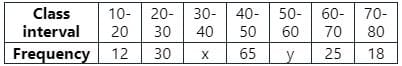Detailed Solution for Test: Measures of Central Tendency - 1 - Question 4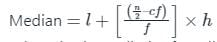Where l = lower limit of median class,

n = number of observations,

h = class size, f = frequency of median class,

cf = cumulative frequency of class preceding the median class.
Calculation: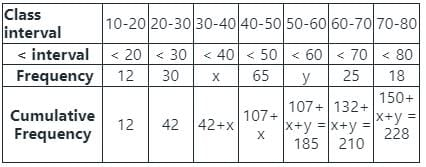From the given table we have formed the above table of cumulative frequency to find the median.

In the table, n = 150 + x + y = 150 + 78 = 228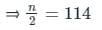which will lie between 42 + x and 107 + x,

therefore the median class: 40 - 50

l = 40, f = 65, cf = 42+x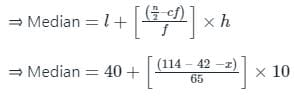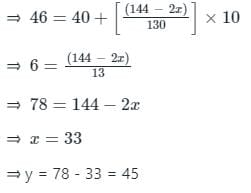Test: Measures of Central Tendency - 1 - Question 5

If mean of 14, 13, 18, 16, k, (k + 3) is 13, then what will be the mean of k, 8, 9, 11, 5, 10, 6?

Detailed Solution for Test: Measures of Central Tendency - 1 - Question 5

The mean of 14, 13, 18, 16, k, (k + 3) = 13 which means

⇒ [14 + 13 + 18 + 16 + k + (k + 3)]/6 = 13

⇒ [ 2k + 64] = 78

⇒ k = 7

Then, the mean of k, 8, 9, 11, 5, 10, 6 is

⇒ [k + 8 + 9 + 11 + 5 + 10 + 6]/7

⇒ [7 + 8 + 9 + 11 + 5 + 10 + 6]/7

⇒ 56/7

⇒ 8

∴ The correct answer is 8

Test: Measures of Central Tendency - 1 - Question 6

What is the mode of 8, 5, 7, 10, 15, 21, 5, 7, 2, 5?

Detailed Solution for Test: Measures of Central Tendency - 1 - Question 6

Mode in a data set is defined as the number that occurs most frequently in a data set.
5 occurred three times, 7 occurred two times, 2 and 21 occurred one time each.
5 is the most repeated number according to the definition mode.

Test: Measures of Central Tendency - 1 - Question 7

Construction of a cumulative frequency table is useful in determining the:

Detailed Solution for Test: Measures of Central Tendency - 1 - Question 7

Median

Cumulative frequency distribution is the sum of the frequency of class and all classes below it in a frequency distribution.

This means we can get cumulative frequency by adding up a value and all of the values that came before it.

Also,

Frequency of any class = cumulative frequency of class - cumulative frequency of preceding class.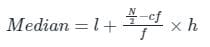l = lower limit of median class interval
cf = cumulative frequency preceding to the median class frequency
f = frequency of the class interval to which median belongs
h = width of the class interval

Test: Measures of Central Tendency - 1 - Question 8

If the mean of 10 numbers is 96 and one of the numbers is 150, then what is the mean of the remaining nine numbers?

Detailed Solution for Test: Measures of Central Tendency - 1 - Question 8

Here, the mean of 10 numbers is 96,

Then, total of the 10 members = 96 × 10 = 960

If one member is 150, then the total of  remaining 9 members = (960 - 150) = 810

Now, the mean of the remaining nine numbers = 810/9 = 90

∴ The correct answer is 90

Test: Measures of Central Tendency - 1 - Question 9

Below are the results of the students in an exam. Find the mode of given results.
90, 80, 77, 86, 90, 91, 77, 25, 45, 35, 66, 69, 65, 43, 65, 75, 43, 90, 89.

Detailed Solution for Test: Measures of Central Tendency - 1 - Question 9

90 occurred thrice. 77, 43 and 65 occurred twice.
In the above data set, 90 occurred three times and other numbers appeared once or twice. So, 90 is the mode of the above data set.

Test: Measures of Central Tendency - 1 - Question 10

Find the median of 4.1, 5.2, 6.3, 3.6, 2.4, 6.4, 4.6

Detailed Solution for Test: Measures of Central Tendency - 1 - Question 10

Concept:

Median: It is the middle value in the given set, while the set is either in increasing or decreasing order.

If the number of observation (n) is odd then median is (n+1)/2th term.

If the number of observation (n) is even then the median is average of nth and (n+1)th term.

Calculation:

Given data are 4.1, 5.2, 6.3, 3.6, 2.4, 6.4, 4.6

Increasing order of given data: 2.4, 3.6, 4.1, 4.6, 5.2, 6.3, 6.4

The number of observation is odd (n = 7)

So, the median is the  (n+1)/2th term or 4th term

Median is 4th term i.e., 4.6

## Statistics for SSC CGL

69 videos|90 docs|16 tests
Information about Test: Measures of Central Tendency - 1 Page
In this test you can find the Exam questions for Test: Measures of Central Tendency - 1 solved & explained in the simplest way possible. Besides giving Questions and answers for Test: Measures of Central Tendency - 1, EduRev gives you an ample number of Online tests for practice

## Statistics for SSC CGL

69 videos|90 docs|16 tests